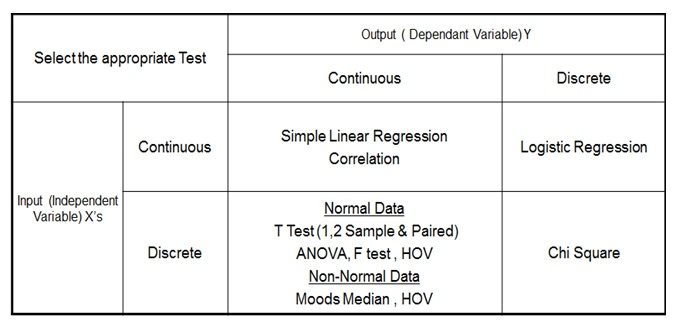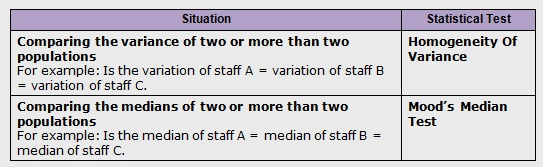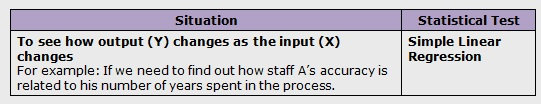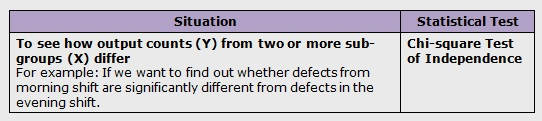# Six Sigma DMAIC Process - Analyze Phase - Hypothesis Testing

In a process, we may face Problem with Centering and/or Problem with Spread. Below diagram will allow us to understand these two problems in detail:Practical Six Sigma Problems that require Hypothesis Testing

Hypothesis testing tells us whether there exists statistically significant difference between the data sets for us to consider that they represent different distributions.

What is the difference that can be detected using Hypothesis Testing?
For Continuous data, hypothesis testing can detect Difference in Average and Difference in Variance. For Discrete data, hypothesis testing can detect Difference in Proportion Defective.

Steps in Hypothesis Testing:
• Step 1: Determine appropriate Hypothesis test
• Step 2: State the Null Hypothesis Ho and Alternate Hypothesis Ha
• Step 3: Calculate Test Statistics / P-value against table value of test statistic
• Step 4: Interpret results – Accept or reject Ho
Mechanism:
• Ho = Null Hypothesis – There is No statistically significant difference between the two groups
• Ha = Alternate Hypothesis – There is statistically significant difference between the two groups
Hypothesis Testing Errors:
Type I Error – P (Reject Ho when Ho is true) = α
In type I Error, we reject the Null Hypothesis when it is true. It is also called as Alpha error or Producer’s Risk.

Type II Error - P (Accept Ho when Ho is false) = β
Similarly, in type II Error, we accept Null Hypothesis when it is false. It is also called as Beta error or Consumer’s Risk.Six Sigma Hypothesis Testing Errors

P Value – Also known as Probability value, it is a statistical measure which indicates the probability of making an α error. The value ranges between 0 and 1. We normally work with 5% alpha risk, a p value lower than 0.05 means that we reject the Null hypothesis and accept alternate hypothesis.

Types of Hypothesis Testing:
We use the following grid to select the appropriate hypothesis test depending on the data types:Types of Six Sigma Hypothesis TestingNormal Continuous Y and Discrete XNon-Normal Continuous Y and Discrete XContinuous Y and Continuous XDiscrete Y and Discrete XSix Sigma Hypothesis Test – Null and Alternate Summary

# We guarantee that Your Free Online Training will make you pass Your Six Sigma Certification Exam!